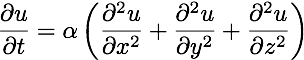## Saturday, January 22, 2011

### Quantized Redshifts. II. The Fourier Series

The Tools
The mathematical tool commonly used in reports of redshift quantization is the power spectral density (PSD) (Wikipedia).  You've seen simple versions of the PSD in the form of a graphic equalizer in computer applications such as iTunes.  Sound engineers use a graphic equalizer to adjust audio power in multiple frequency bands (Wikipedia).  While the PSD is an excellent tool for identifying well-defined frequencies, broadband signals which cover many frequencies, require considerably more effort to interpret.  To understand this requires a bit more exploration of just what the PSD is and does.

History
Charles Fourier was the father of what we now call the set of transformations that bear his name.  Why did Fourier develop these transformations?  Fourier was working on the problem of heat transfer, examining solutions of the equation (Wikipedia).For very simple cases of conduction between two planar surfaces, the solution to the equation seemed intractable.   But Fourier decided to approach the problem in a standard reductionist way - can the big problem that is unsolvable be broken down into a set of simpler problems that can be solved by the existing techniques.  Because the heat transfer equation was a differential equation of 2nd-order, one possible set of simpler solutions might be a weighted sum of sines and cosines.  Fourier explored the idea that any function f(x) in a range of position, x, from -L to +L, might have an alternative representation as a sum of sines and cosines.  In mathematical notation, this is written,
where n is an integer ranging from one to infinity.  Fourier found that this equation would be true if the coefficients, an, and bn, were given by the integrals:

The technique provided the means for Fourier to re-write the heat transport equation into a sum of 2nd-order differential equations where the individual terms did have simple solutions.  Then the method would allow him to recombine the simple solutions into the complete solution to the problem.

Caveat: Care should be exercised as some sources define a0 and the normalization of an and bn different than defined here.  So long as a consistent set of series and coefficients are used, there should be no problem.

Fourier Series: Frequencies Everywhere!
The technique would prove to be incredibly powerful, and would open the door to the more generalized methods of orthogonal functions (wikipedia).  These techniques would become very important for solving the more complex equations that would be developed for electromagnetism (Maxwell's Equations) and quantum mechanics (Schrodinger Equation).

But the Fourier series has another implications important for our story

All functions of a finite range could be expressed as a sum of sine and cosine waves.  The cosine wave is equivalent to a sine wave shifted in phase by 90 degrees (pi/2 radians).

Caveat: I say 'All functions', but there are some limitations, called Dirichlet conditions (Wikipedia).  However, it is easy to see that in a practical sense, it means that almost any function that can represent a physical system will have a Fourier Series.

Let's look at a function and its Fourier series.

We'll start with a square wave (Wikipedia).  The square wave can be expressed mathematically as

and graphically, we'll show two periods of the wave.

From the 'recipe' above, we can compute the Fourier coefficients for this profile, a & b:

We plot the amplitude of these coefficients, using different colors for the sine and cosine components.  The dot represents the actual amplitude of the coefficient (vertical axis) for a given integer, n (the horizontal axis).

In this example, we see that for the cosine terms in blue, only the first coefficient, a0, is non-zero.  All other cosine terms are zero.  The sine terms, in red, are very different - we see that the even frequencies are zero and the odd frequencies are non-zero.

As a check on our result, and to illustrate that the Fourier series really does work, we can plug these coefficients into the Fourier series and add them up and see how they compare to the original function.  To examine how the result behaves when we include more terms, we'll use multiple colors to distinguish the curves with a different number of terms.  The black curve is the original square wave.  The cyan curve is constructed from only two terms, red includes four terms, blue includes eight terms, and green is 32 terms..Click to Enlarge

Notice that the more terms we include, the more closely the series value matches the original function in black.  There is only one discrepancy, and that is the Gibbs phenomena (wikipedia) visible at the top of the square where it turns down.  This occurs at strong discontinuities in the function.  The more terms we include, that overshoot will get narrower in width, but it is an unfortunate artifact of the discontinuity in the square wave.

Next Weekend: The PSD connection